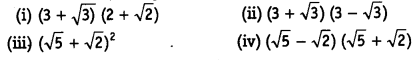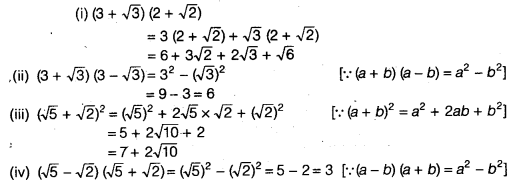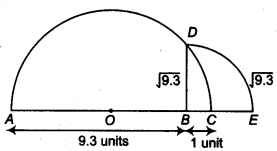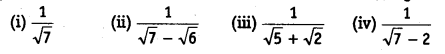## NCERT Solutions for Class 9 Maths Chapter 1 Number Systems Ex 1.5

NCERT Solutions for Class 9 Maths Chapter 1 Number Systems Ex 1.5 are part of NCERT Solutions for Class 9 Maths. Here we have given NCERT Solutions for Class 9 Maths Chapter 1 Number Systems Ex 1.5.

 Board CBSE Textbook NCERT Class Class 9 Subject Maths Chapter Chapter 1 Chapter Name Number Systems Exercise Ex 1.5 Number of Questions Solved 5 Category NCERT Solutions

## NCERT Solutions for Class 9 Maths Chapter 1 Number Systems Ex 1.5

Question 1.
Classify the following numbers as rational or irrational.Solution:
(i) Irrational ∵ 2 is a rational number and √5 is an irrational number.
∴ 2.√5 is an irrational number.
(∵The difference of a rational number and an irrational number is irrational)
(ii) 3 + $$\sqrt{23}$$ – $$\sqrt{23}$$ = 3 (rational)
(iii) $$\frac { 2\sqrt { 7 } }{ 7\sqrt { 7 } }$$ (rational)
(iv) $$\frac { 1 }{ \sqrt { 2 } }$$(irrational) ∵ 1 ≠ 0 is a rational number and $$\sqrt{2}$$≠ 0 is an irrational number.
∴ $$\frac { 1 }{ \sqrt { 2 } }$$ is an irrational number. 42
(∵ The quotient of a non-zero rational number with an irrational number is irrational).
(v) 2π (irrational) ∵ 2 is a rational number and π is an irrational number.
∴ 2x is an irrational number. ( ∵The product of a non-zero rational number with an irrational number is an irrational)

Question 2.
Simplify each of the following expressionsSolution:Question 3.
Recall, π is defined as the ratio of the circumference (say c) of a circle to its diameter (say d). That is π = $$\frac { c }{ d }$$. This seems to contradict the fact that n is irrational. How will you resolve this contradiction?
Solution:
Actually $$\frac { c }{ d }$$ = $$\frac { 22 }{ 7 }$$,which is an approximate value of π.

Question 4.
Represent $$\sqrt{9.3}$$ on the number line.
Solution:
Firstly we draw AB = 9.3 units. Now, from S, mark a distance of 1 unit. Let this point be C. Let O be the mid-point of AC. Now, draw aemi – circle with centre O and radius OA. Let us draw a line perpendicular to AC passing through point B and intersecting the semi-circle at point D.∴ The distance BD = $$\sqrt{9.3}$$
Draw an arc with centre B and radius BD, which intersects the number line at point E, then the point E represents $$\sqrt{9.3}$$ .

Question 5.
Rationalise the denominator of the followingSolution:We hope the NCERT Solutions for Class 9 Maths Chapter 1 Number Systems Ex 1.5, help you. If you have any query regarding NCERT Solutions for Class 9 Maths Chapter 1 Number Systems Ex 1.5, drop a comment below and we will get back to you at the earliest.